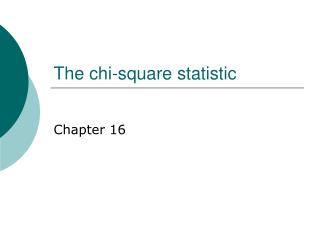DownloadDownload PresentationThe chi-square statistic

# The chi-square statistic

Télécharger la présentation## The chi-square statistic

- - - - - - - - - - - - - - - - - - - - - - - - - - - E N D - - - - - - - - - - - - - - - - - - - - - - - - - - -
##### Presentation Transcript

1. The chi-square statistic Chapter 16

2. The tests we’ve used so far… • All involve one quantitative variable • One sample t-test: compare mean of sample on a quantitative variable to some meaningful value • Independent samples t-test: compare means of two groups on a quantitative variable • Paired samples t-test: compare pairs of scores on a quantitative variable

3. What if you don’t have a quantitative variable? • For instance, if you want to know if more males or females volunteer to participate in psychology studies • Or if you want to know if the percentage of males who prefer blue to pink is different from the percentage of females who prefer blue to pink • No quantitative variable •  can’t estimate parameter in the population •  need to use a non-parametric statistic

4. Two different situations • One qualitative variable, and want to compare which group people are most likely to belong to • Two qualitative variables, and want to know whether the percentages in each category for one group of people differ from the percentages in each category for another group of people

5. The first situation • Are people more likely to belong to one group than another? • Null hypothesis = nothing’s going on – people should be equally likely to belong to each group or category • Question: How well does this model fit the data •  Chi-square test for goodness of fit

6. How to test this • Like all statistical tests, compare the data that you have with what you would have if the null hypothesis was true • Data you have = observed frequencies for each group • What you expect to see if the null were true = expected frequencies for each group • Larger the difference between these, the bigger the chi square statistic, and the smaller the p value

7. Quantifying this • For each category: • 1. count the number of cases observed in that category • 2. subtract the number of cases you would expect to see in that category if the null hypothesis were true • 3. square this difference • 4. divide by the number of cases you would expect to see in that category if the null hypothesis were true • 5. add up across categories • If the null hypothesis is true, this should be close to 0

8. How big is big enough? • Compare calculated chi square value to critical chi square value • If calculated value is larger, reject the null, since p is smaller than alpha • To get critical chi square, need df • df = number of categories minus 1

9. Telling the world • Similar format to t • c2 (df, n = x) = calculated chi square value, p information

10. Another situation for the goodness of fit test • If, based on prior research and theory, you have reason to expect that the distribution of people across categories will follow a particular pattern, you can use these values as the expected values • E.g., if there are 60% females in the population, it wouldn’t make sense to compare the distribution of genders volunteering for a study to a 50-50 breakdown

11. The second type of chi square • You have different types of participants (e.g., males/females, people with different majors) • You want to know whether which type of participant they are is independent of some other variable • E.g., is gender independent of color preference? •  chi square test for independence

12. Null hypothesis • Nothing’s going on •  the two variables are independent of each other •  if, overall, 25% of people like pink, the people who like pink should be equally likely to be male and female •  the breakdown of categories for the entire sample should be the same as the breakdown of categories for sub-groups of the sample

13. Calculating the test for independence • Exactly the same formula as the test for goodness of fit • For each category: • 1. observed frequency minus expected frequency, squared • 2. divided by expected frequency • 3. add up across categories

14. How big is big enough? • Need critical chi square value • df = df one variable * df other variable • df = (number categories – 1) * (number categories – 1)

15. Effect size still matters • A chi square could be significant, or not significant, due to sample size •  need to know how much of something is going on •  phi: square root of (chi squared divided by number of participants)